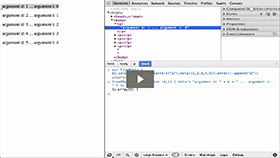Using Data Bound to DOM Elements
Duration: 11:57
Part of Course: Introductory D3 Course
This Lesson is for subscribersUnlock this lesson NOW!
• Get expert advice on Using Data Bound to DOM Elements
• Start mastering D3.js right now!

Takeaways:

• The D3 Data Operator joins a specified array of data with the current selection
• If the D3 Data Operator is called without an arguments, then it returns the __data__ property for each element in the selection
• The D3 Text Operator is a shortcut for setting the text content of all selected elements to the specified value
• If the value passed into the D3 Text Operator is a constant, then all the elements in the selection are given the same text content
• If the value passed into the D3 Text Operator is a function, then the function is evaluated for each element in order they exist in the selection
• Because JavaScript functions are first-class objects, we can pass JavaScript functions into D3 Operators
• If the value passed into the D3 Text Operator is a function, then the value returned from the function evaluation of each selection element is set as the text content for that specific element.
• Almost all D3 operators give you three variables you can use with functions passed into the operator - d (for __data__), i (for index of element in the selection), this (which refers to the actual selection element), and j (if you are working with nested data)

Resources:

D3 Video Tutorial Lessons:

This Transcript is for subscribers.Unlock this D3 Tutorial NOW!
• Get expert advice on Using Data Bound to DOM Elements